# Decomposing & Working with Time Series (Time Series #1)

In this post/Jupyter Notebook we’ll look at Time Series and theory surrounding them.
machine-learning
timeseries
workshop
Author

Hampus Londögård

Published

March 11, 2022# [CA]: Time Series #1 - Decomposing & Working with Time Series

`CA=Competence Afternoon`

This CA is originally found on kaggle.com/lundet/.., as we entered a competition to predict cryptocurrency prices - G-Research Crypto Forecasting.

N.B. This blog/notebook is adapted into a Jupyter notebook that’s easier to replicate, that is we don’t use the Kaggle API + GBs of data that was required for said competition.

Moving on to the content! 🤓

## Time Series Concepts

Time Series has some important attributes that are unique compared to other data types such as `Text`, `Image` and `Tabular`.

Time Series can be decomposed into multiple other time series that together compose the decomposed one (composition baby!).

Trend Seasonality Combined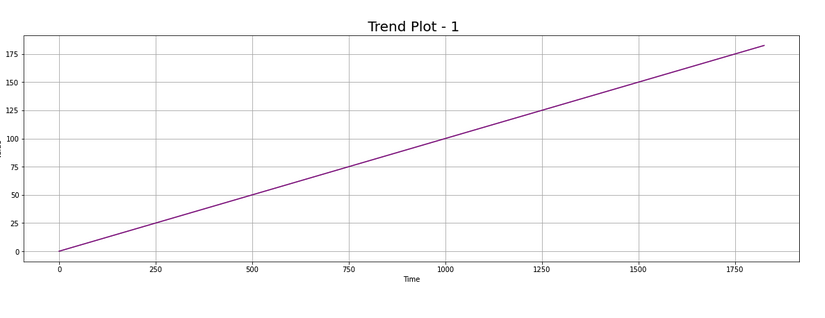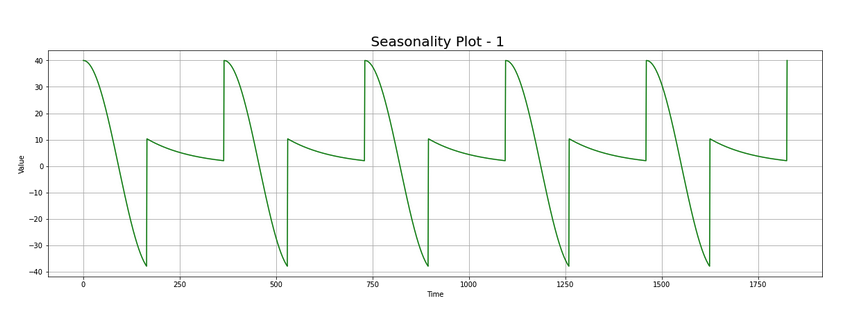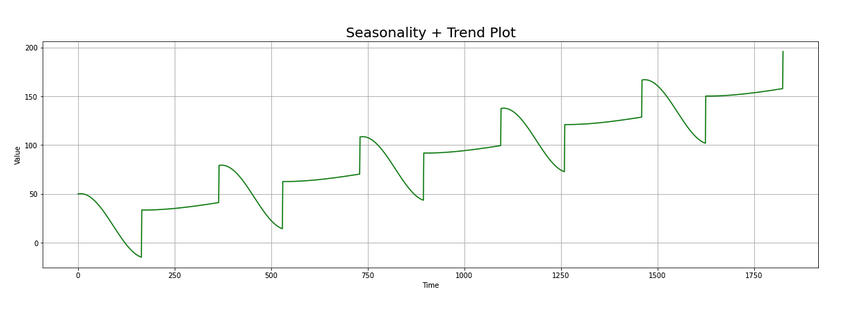As shown above we can build a time series out of a `Trend` and `Seasonality`, that means we can also decompose the `Combined` into `Trend` and `Seasonality`.

This can be done in a few ways, mostly either through a `additive` or `multiplicative` decomposition.

• Additive means that if we add `Trend` and `Seasonality` together we create `Combined`.
• Multiplicative means that if we multiply `Trend` and `Seasonality` together we create `Combined`.

But these two are not enough to compose a full time series, usually we have noise too.

Noise Trend+Seasonality+Noise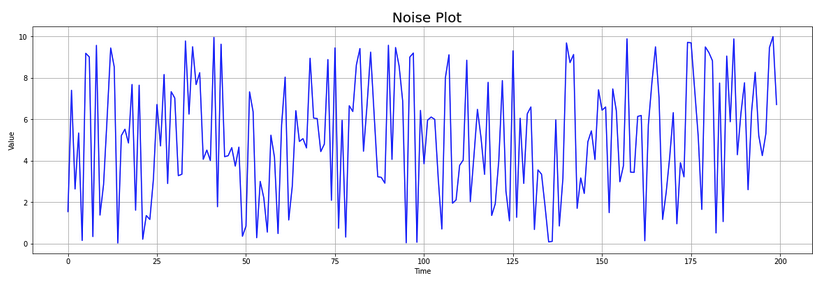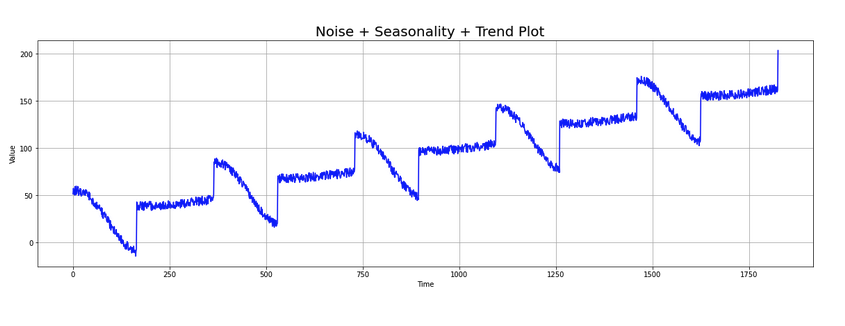It seems we’re onto something. But still it’s not really how we usually see time series!

What else is left? Autocorrelation!

Autocorrelation is a correlation between two observation at different time steps, if values separated by an interval have an strong positive or negative correlation it’s indicated that past values influence the current value.

To keep it simple, if a time series is autocorrelated it’s new state has a correlation with a earlier step.

Autocorrelation Trend+Autocorrelation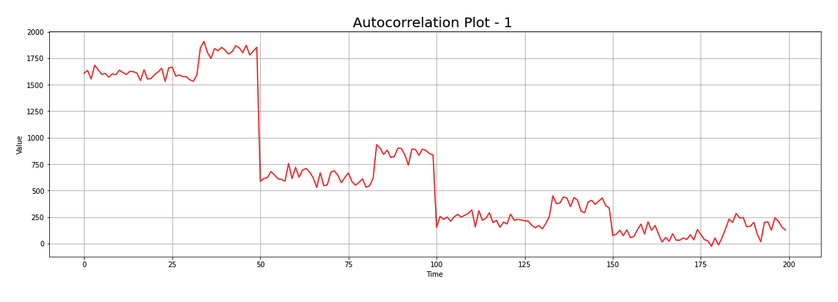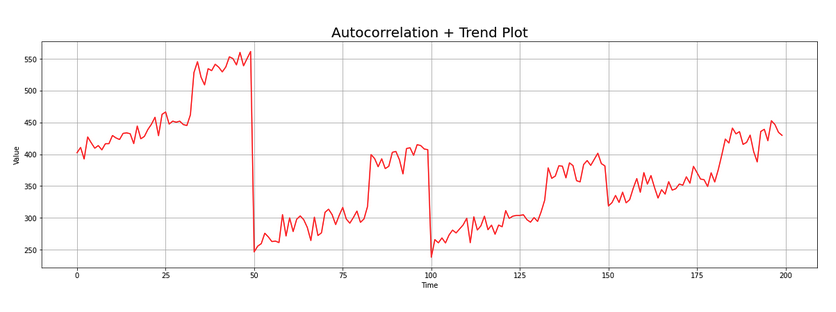Trend+Seasonality+Autocorrelation Trend+Seasonality+Autocorrelation+Noise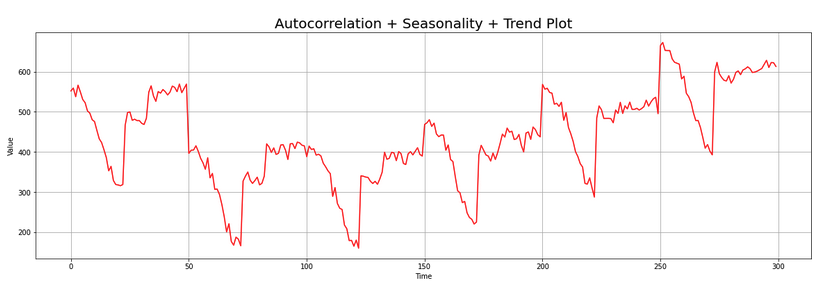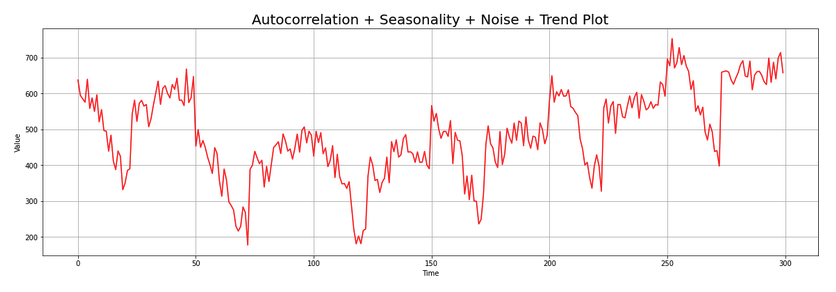And there we go! 😎

We got a legit timeseries, pat yourself on your back and be proud. We did it.

Let’s get started with real data… 🧑‍💻

### Installation & Importing

We need to have libraries which allows us to simplify operations and focus on the essential.

• `pandas` is a `DataFrame` library that allows you to visualize, wrangle, and investigate data in different ways
• `numpy` is a math library which is implemented in `C` and `Fortran` and combined with vectorized code it’s incredibly fast!
• This is key in Python as Python is a really slow language.
• `pandas_datareader` gives us easy access to different data formats, directly in `pandas`
• `plotly` gives your plots the next level interactivity that you’ve always wanted
``````from IPython.display import clear_output
!pip install plotly

clear_output()``````

And importing our libraries

``````import pandas as pd # data processing, CSV file I/O (e.g. pd.read_csv)
import numpy as np  # linear algebra

from datetime import datetime``````

Using `pandas_datareader` we can query multiple API:s, and among them Yahoo.
Yahoo as a financial API that follows stocks, currencies, cryptocurrencies and much more!

Let’s see what we can do.

``````df = pdr.get_data_yahoo('BTC-USD', start=datetime(2020, 1, 1), end=datetime(2022, 1, 1))
High Low Open Close Volume Adj Close
Date
2020-01-01 7254.330566 7174.944336 7194.892090 7200.174316 18565664997 7200.174316
2020-01-02 7212.155273 6935.270020 7202.551270 6985.470215 20802083465 6985.470215
2020-01-03 7413.715332 6914.996094 6984.428711 7344.884277 28111481032 7344.884277
2020-01-04 7427.385742 7309.514160 7345.375488 7410.656738 18444271275 7410.656738
2020-01-05 7544.497070 7400.535645 7410.451660 7411.317383 19725074095 7411.317383

The data seems great, but one should always validate it visually and programmatically.

Plotting can be done in multiple ways, let’s start using the `pandas` way. 🐼

pandas/docs/plot
Make plots of Series or DataFrame.
Includes options like `x`, `y`, `kind` (e.g. `line`, `bar` or `density`) and much more.

``df['Close'].plot()``
``<AxesSubplot:xlabel='Date'>``Let’s add some interactivity to this, and plot `Low`/`High` on top of that!
Super simple using `plotly` as the backend! 😎

``df[['Close', 'High', 'Low']].plot(backend='plotly')``
Show plotly chart (as it’s invisible in blog-mode)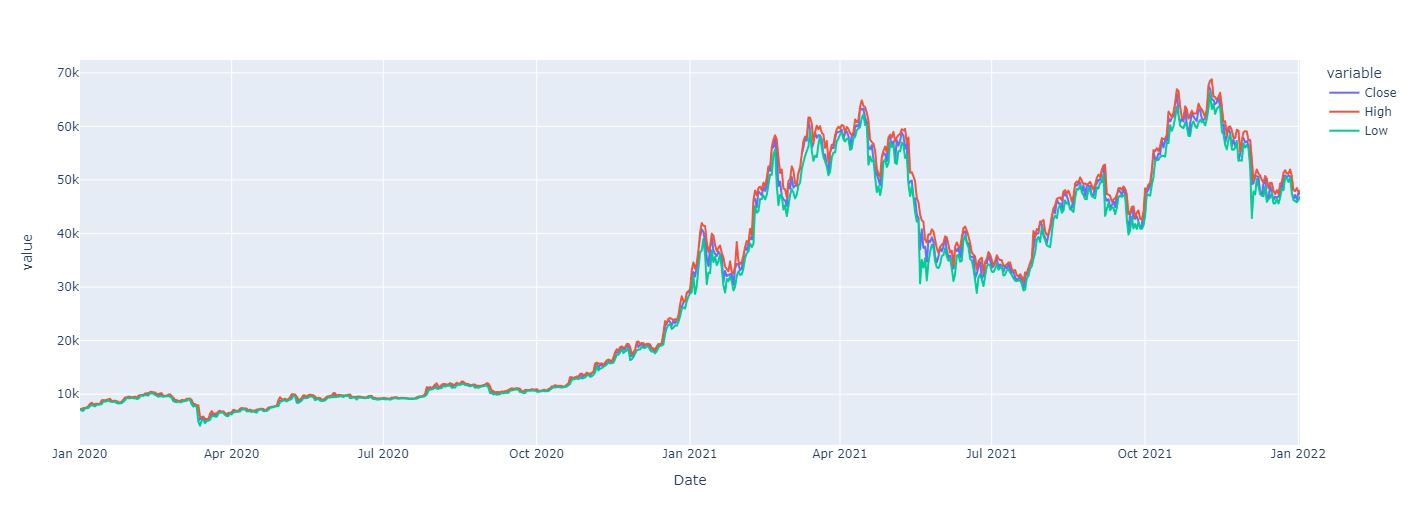You can also plot a candle-stick chart by using

``````import plotly.graphical_objects as go

fig = go.Figure(data=[go.Candlestick(x=df.index,
open=df['Open'],
high=df['High'],
low=df['Low'],
close=df['Close'])])``````

The data does look nice, but we need to figure if there’s any issues in the data.
Graphs might be missing some values that are not clearly visible as there’s so much data. Our final data validation should always be programatical.

## Data Validation

Validating that data makes sense and that there’s no errors is very important when you’re building models. Having outliers, errors and other things can lead to some really weird behaviour.

There’s a few tools we can use:

`pd.DataFrame.isna`:
Detect missing values. Return a boolean same-sized object indicating if the values are NA. NA values, such as None or numpy.NaN, gets mapped to True values.
💡The reverse, `notna` also exists.

``df.isna().head()``
High Low Open Close Volume Adj Close
Date
2020-01-01 False False False False False False
2020-01-02 False False False False False False
2020-01-03 False False False False False False
2020-01-04 False False False False False False
2020-01-05 False False False False False False

Can we we make this more readable?
Yes! Using `.any()`, or even ‘cheating’ using `.sum()`

``df.isna().any()``
``````High         False
Low          False
Open         False
Close        False
Volume       False
dtype: bool``````
``df.isna().any().any() # 👀``
``False``

LGTM ✅

Next up: Validating that there’s no missing days

This can be done in multiple ways, but for now I choose to use `.diff`.

`pd.DataFrame.diff`: First discrete difference of element.
Bonus: Diff can also handle `periods` and `axis` arguments, `period` being how far to diff.

``df.index.to_series().diff().dt.days.head()``
``````Date
2020-01-01    NaN
2020-01-02    1.0
2020-01-03    1.0
2020-01-04    1.0
2020-01-05    1.0
Name: Date, dtype: float64``````

Ok, so we’ve got a series. Try to validate that no `diff` is greater than 1 day using a broadcasted/vectorized operation.

hint: `pandas` automatically broadcast operations by operator overloading, e.g. `>`, `+` etc

I think we can call quits on the validation part for now.

Let’s move on to different ways we can format the data, a common format is `LogReturn`.

## Transforming Data

Because data is very different moving in time we wish to normalize the data somehow. This can be done in multiple ways, some common ways are:

1. `pd.DataFrame.diff` which takes the difference between x_1 and x_2
• The negative aspect of this is that the difference is still not scaled
1. `pd.DataFrame.pct_change` which validates the % difference
2. `LogReturn` which is the logarithmic return between each time step (x_1, x_2, ..).
3. Apply a `Scaler` which scales the data somehow
• Can be `MinMaxScaler` which scales `Min` and `Max` to (0,1) or (-1,1)
• Can be a `MeanScaler` which scales the data to have a mean of 0.

… and more.

We’ll start of with `LogReturn` which is common in forecasting of stocks.

``````def log_return(series, periods=1):
return np.log(series).diff(periods=periods)``````
``````df['LogReturn'] = log_return(df['Close'])
``````Date
2020-01-01         NaN
2020-01-02   -0.030273
2020-01-03    0.050172
2020-01-04    0.008915
2020-01-05    0.000089
Name: LogReturn, dtype: float64``````

Because `.diff` takes the diff with the next element you’ll end up with a `NaN`, as such we wish to remove the first element.

``````df = df[1:]
``````Date
2020-01-02    6985.470215
2020-01-03    7344.884277
2020-01-04    7410.656738
2020-01-05    7411.317383
2020-01-06    7769.219238
Name: Close, dtype: float64``````
``df['LogReturn'].plot()``
``<AxesSubplot:xlabel='Date'>``Looks nice.

For now we’ll leave it here and move on to looking at `Correlation`.

## Data Analysis: Correlation

``````df = pdr.get_data_yahoo(['BTC-USD', 'ETH-USD'], start=datetime(2020, 1, 1), end=datetime(2022, 1, 1))
Attributes Adj Close Close High Low Open Volume
Symbols BTC-USD ETH-USD BTC-USD ETH-USD BTC-USD ETH-USD BTC-USD ETH-USD BTC-USD ETH-USD BTC-USD ETH-USD
Date
2020-01-01 7200.174316 130.802002 7200.174316 130.802002 7254.330566 132.835358 7174.944336 129.198288 7194.892090 129.630661 18565664997 7935230330
2020-01-02 6985.470215 127.410179 6985.470215 127.410179 7212.155273 130.820038 6935.270020 126.954910 7202.551270 130.820038 20802083465 8032709256
2020-01-03 7344.884277 134.171707 7344.884277 134.171707 7413.715332 134.554016 6914.996094 126.490021 6984.428711 127.411263 28111481032 10476845358
2020-01-04 7410.656738 135.069366 7410.656738 135.069366 7427.385742 136.052719 7309.514160 133.040558 7345.375488 134.168518 18444271275 7430904515
2020-01-05 7411.317383 136.276779 7411.317383 136.276779 7544.497070 139.410202 7400.535645 135.045624 7410.451660 135.072098 19725074095 7526675353

And retrieving only the `Close` to have something to compare.

``````df = df['Close']
Symbols BTC-USD ETH-USD
Date
2020-01-01 7200.174316 130.802002
2020-01-02 6985.470215 127.410179
2020-01-03 7344.884277 134.171707
2020-01-04 7410.656738 135.069366
2020-01-05 7411.317383 136.276779

Let’s validate the correlation, e.g. how our values correlate to each other!

``df.corr().style.background_gradient(cmap="Blues")``
Symbols BTC-USD ETH-USD
Symbols
BTC-USD 1.000000 0.903202
ETH-USD 0.903202 1.000000

The correlation looks pretty high… We can use `seaborn` to show even better data by adding the plots.

``````import seaborn as sns

sns.pairplot(df)``````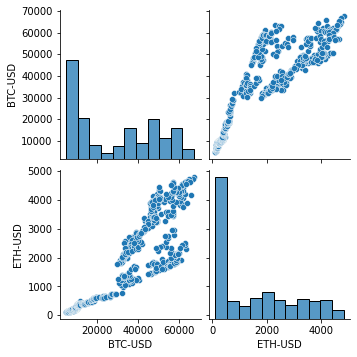Indeed, looks very correlated! 🤩

What conclusions can we take from the above chart? Cryptocurrencies using daily data are indeed correlated, that is ETH prices depends on BTC and v.v.

This blog is getting close to its end, and as such we won’t go further in depth of this.
For the reader an excercise would be to predict the BTC price depending on ETH, which should be possible based on this correlation. We’ll go further into this later.

## Data Analysis: Decomposition

Decomposing Time Series means that we try to find seasonality, trends and other things. This can be done using `statsmodels` which is a very impressive library that originally was done in `R` but now exists in Python.

``````from statsmodels.tsa.seasonal import seasonal_decompose

res = seasonal_decompose(df['BTC-USD'])
res.plot()``````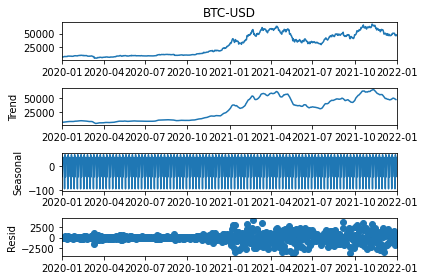Looks nice and dandy! But we can modify this further by adding `model` and `freq` parameter to validate how it looks by decomposing either through `model=multiplicative` or `additive`, and updating the `freq` (`period` in the newest version) to decompose it based on different periods.

``````res = seasonal_decompose(df['BTC-USD'], model='additive', period=365) # Try weekly or monthly decomposition.
res.plot()``````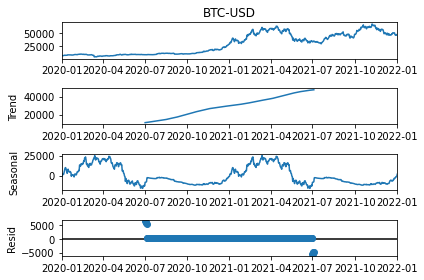We’re now looking at our final data analysis step, Fast Fourier Transform, A.K.A. FFT!

## Data Analysis: FFT

We’ll also add an Fast Fourier Transform which can show which frequencies the data “resets” at.

``````import numpy as np
import matplotlib.pyplot as plt

fft = np.fft.rfft(df['BTC-USD'])

plt.step(range(len(fft)), abs(fft))

plt.xscale('log')
plt.xlim([0.1, max(plt.xlim())])
plt.xticks([1, 365.2524], labels=['1/Year', '1/day'])
_ = plt.xlabel('Frequency (log scale)')``````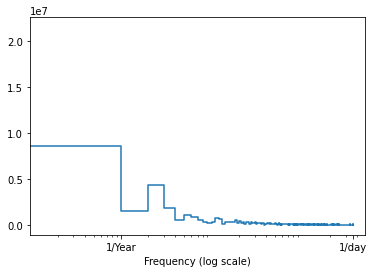That’s it for this time. Make sure to view part 2 if you want to start predicting some data, and in part 3 we’ll do the final prediction where we’ll look at different forecast horizons!